PURE BENDING 2

# 1PURE BENDING 2

## 1.1 Problem definition

Determine the deflections and bending stresses at the middle of the carbon steel beam.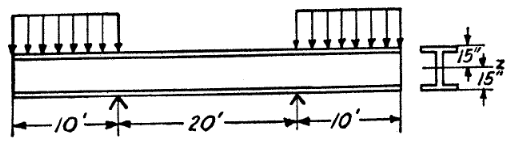Figure 1: Model by Timoshenko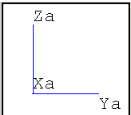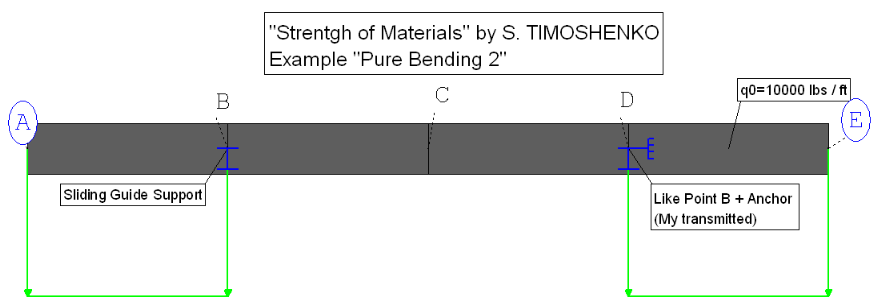Figure 2: Rohr2 Model

## 1.2 References (Timoshenko)

S. Timoshenko, Strength of Material, Part I, Elementary Theory and Problems, 3rd Edition, D. Van Nostrand Co., Inc., New York, NY, 1956, Chapter 4.23, pg. 95.

The thirty inch high carbon structure is stressed at both ends by a constant line load. The following values are given:

• Length of several chapter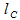• Total length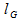• Constant line load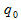• Moment of Inertia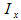• Max. fiberdistance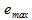• Modulus of Elasticity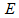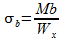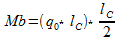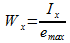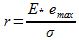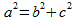Where:

 Variable Description Unit Used Value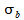Maximum stress lbs/ inchÂ² 9836,066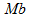Bending Moment ft lbs 6000000,0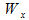Section Modulus inchÂ³/cmÂ³ 610,0/9996,10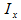Moment of Inertia inch4/cm4 9150,0/380853,25Max. fibre distance inch 15,00Chapter length ft 10,00Total length ft 40,00Line load lbs/ ft 10000,00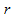Radius of curvature inch 45750,00Modulus of Elasticity lbs/ inchÂ² 30000000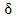Deflection inch 0,01574

Table 1: Overview of the used variables

## 1.3 Model description (ROHR2)

The system consists of four equal long bars (lC= 10 ft â‰™ 120 inch). For this model it necessary to generate a new profile (IPB30â€œ see figure 4) with the given moment of inertia of 9150,0 inch4. The section parameters of the profile are written in the profile database (see figure 3).The beam is supported by two simple supports. At point B the torsion and axial displacement is fixed to avoid a singular system: At both sides, between end and boundary condition, constant line loads are applied. The line load were increased by a factor of 100 to increase the number of significant digits in the output. In the Lc Load1 the gravitational acceleration is not taken into account.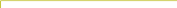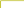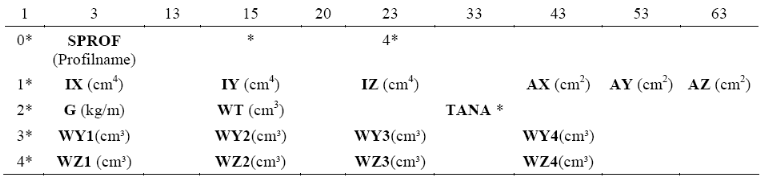Figure 3: Section parameters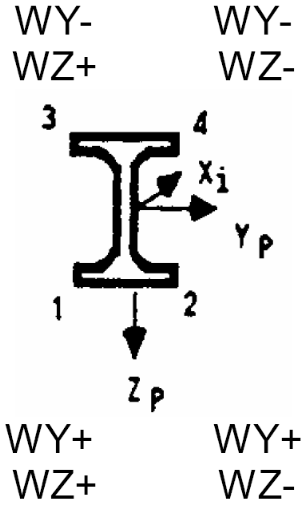Figure 4: Declaration the axes of an I- profile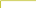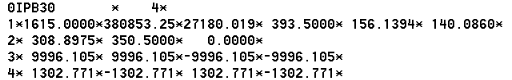Figure 5: Input from the PROFDS.r2u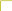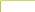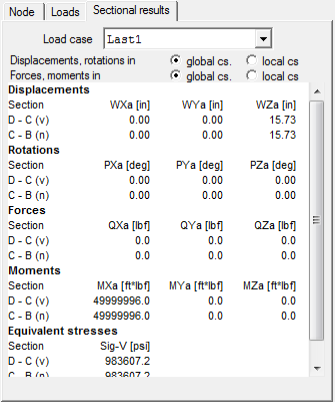Figure 8: Results for the increased load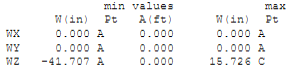Figure 7: Î´ from the Load case 1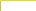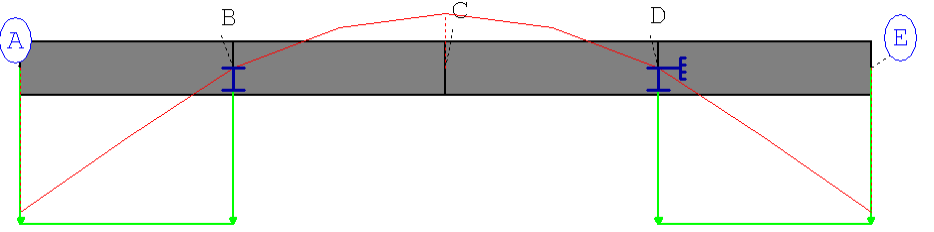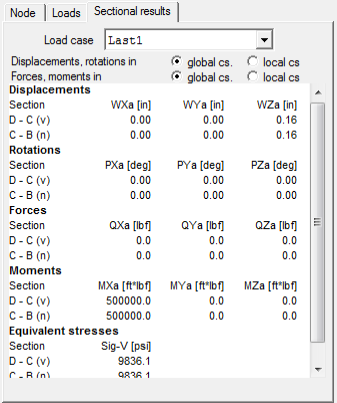Figure 6: Sectional results (equivalent stress) a point C

## 1.4 Result comparisons

 Value Length [inch] Reference (Timoshenko) [lbs/inchÂ²] Rohr2 [lbs/inchÂ²] Difference [%]480,0 9836,07 9836,1 <0,01

Table 2: Comparison of the stress at the middle of the system

 Value Length [inch] Reference (Timoshenko) [inch] Rohr2 [inch] Difference [%]480,0 0,15738 0,15726 <0,08

Table 3: Comparison of the max. deflection

## 1.5 Conclusion

The results are perfectly matching the reference values.

## 1.6 Files

R010_inch.r2w

R010_mm.r2w

R2_stresses_10.xls

PROFDS.r2u

SIGMA Ingenieurgesellschaft mbH www.rohr2.com